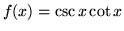Sample Midterm Exam 2

Question 1 (10). Find the derivative of the following functions:
a.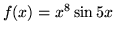b.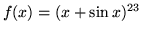c.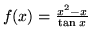d.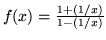e.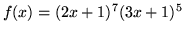Question 2 (15). a. State the definition of the derivative of a function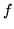at a point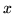.

b. Use the definition of the derivative to compute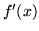for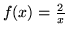Question 3 (10). Find all the vertical and horizontal asymptotes of the graph of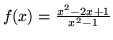Question 4 (20). For each of the following, either find the limit or state that "no limit exists" and briefly explain why. Show work used to get your answer.

a.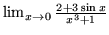b.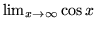c.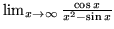d.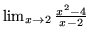.

Question 5 (10). For the function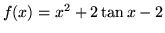a. Find the equation of the tangent line to the graph of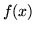at the point (0,-2).

b. Show that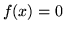at some point.

Question 6 (10) a. State the precise definition of what is meant by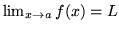.

Use the precise definition of the limit to prove that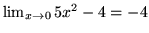.

Question 7 (5) Give an example of a functionwhich is continuous at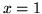but not differentiable at.

Question 8 (5) Supposeand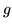are functions and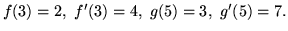Where can you calculate the derivative of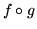? What is it equal to?

Question 9 (5) Let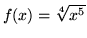. Find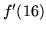.

Question 10 (10). Find an anti-derivative of the following functions:
a.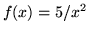b.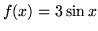c.